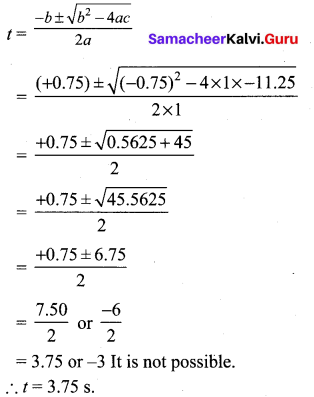## Tamilnadu Samacheer Kalvi 10th Maths Solutions Chapter 3 Algebra Ex 3.11

10th Maths Exercise 3.11 Samacheer Kalvi Question 1.
Solve the following quadratic equations by completing the square method
(i) 9x2 – 12x + 4 = 0
(ii) $$\frac{5 x+7}{x-1}$$ = 3x + 2
Solution: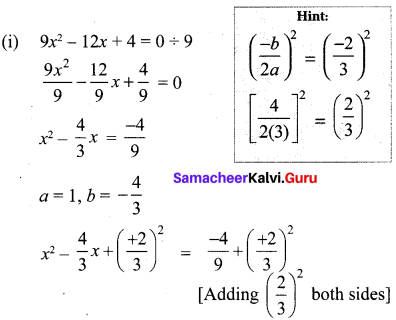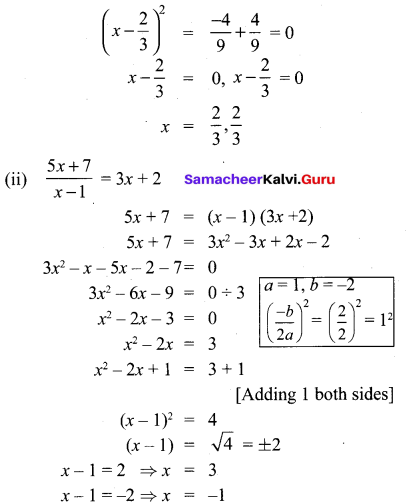Ex 3.11 Class 10 Samacheer Question 2.
Solve the following quadratic equations by formula method
(i) 2x2 – 5x + 2 = 0
(ii) $$\sqrt{2} f^{2}$$ – 6f + $$3 \sqrt{2}$$
(iii) 3y2 – 20y – 3 = 0
(iv) 36y2 – 12 ay + (a2 – b2) = 0
Solution:
(i) 2x2 – 5x + 2 = 0
The formula for finding roots of a quadratic equation ax2 + bx + c = 0 is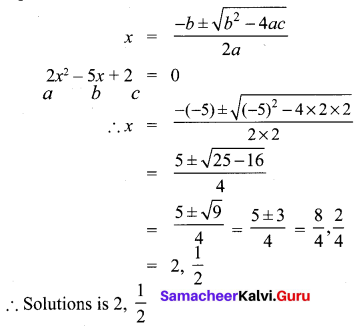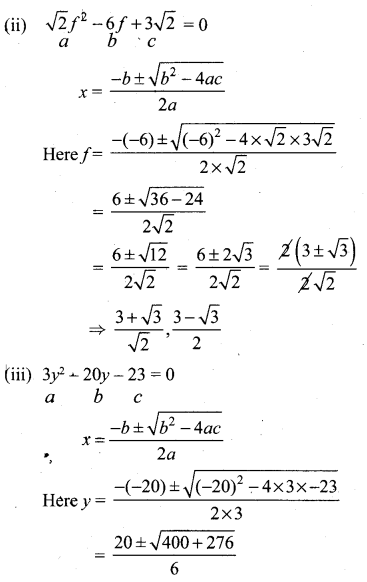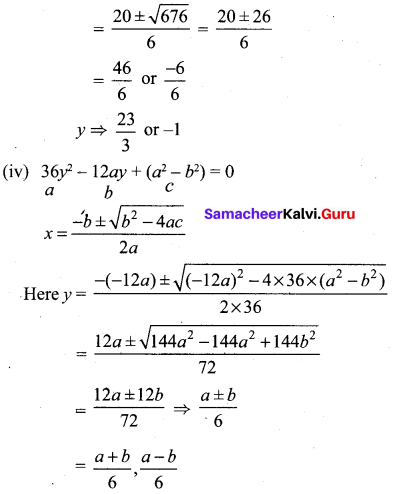Exercise 3.11 Class 10 Samacheer Question 3.
A ball rolls down a slope and travels a distance d = t2 – 0.75t feet in t seconds. Find the time when the distance travelled by the ball is 11.25 feet.
Solution:
Distance d = t2 – 0.75 t,
Given that d = 11.25 = t2 – 0.75 t.
t2 – 0.75t – 11.25 = 0